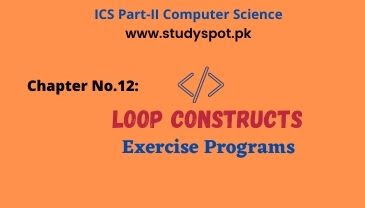# Loop Constructs Exercise ProgramsLoop Constructs Exercise Programs. This post is for the students of Computer ICS Part 2 | Computer Science 2nd Year Chapter No.12 Loop Constructs. Find the simple and easy solution of exercise programs in C language for the final exams.

We tried our best to provide the most simple solution of exercise questions in C language. This post covers the topics i.e., introduction to repetition | loop, while loop, do-while loop, for loop, nested loop, sentinel value, sentinel loop, and conditional loop in C programming language.

If you have any query or suggestion, please feel free to ask us in comments for further betterment and stay linked with us for more multiple choice questions (MCQs) | Quizzes, short questions and answers, and solution of exercise programs.

## Chapter No.12: Loop Constructs

### Exercise Programs

Q.9: Write a program that inputs a number and displays the message “Prime number” if it is a prime number, otherwise displays “Not a prime number”.

To display the message “Prime number” if it is a prime number, otherwise displays “Not a prime number”, follow the code below:

#include<stdio.h>

#include<conio.h>

void main(void)

{

int n,i;

printf("Enter any number:");

scanf("%d",&n);

for(i=2;i<n;i++)

{

if(n%i==0)

{

printf("\n\t Not a prime number!");

goto lab;

}

}

printf("\n\t Prime number!");

lab:

printf("\n");

}

Q.10: Write a program that displays the first 15 even numbers.

To display the first 15 even numbers, follow the code below:

#include<stdio.h>

#include<conio.h>

void main(void)

{

int i=2;

printf("First 15 Even Numbers:");

while(i<=30)

{

printf("\n%d",i);

i=i+2;

}

}

Q.11: Write a program that inputs a number, and displays its table according to the following format:

Suppose the number entered is 5, the output will be as follows:

5 * 1 = 5

5 * 2 = 10

5 * 3 = 15

.

.

.

5 * 10 = 50

To display a table for the above mentioned format, follow the code below:

#include<stdio.h>

#include<conio.h>

void main(void)

{

int n,i;

printf("Enter any number:");

scanf("%d",&n);

for(i=1;i<=10;i++)

{

printf("%d*%d=%d\n",n,i,n*i);

}

}

Q.12: Write a program using do-while loop that repeatedly prompts for and takes input until a value in the range 0 through 15 inclusive is input. The program should add all the values before exiting the loop and displays their sum at the end:

To fulfill the requirements mentioned on the above statement, follow the code below:

#include<stdio.h>

#include<conio.h>

void main(void)

{

int n,sum=0;

do

{

printf("Enter any number:");

scanf("%d",&n);

sum=sum+n;

}while(n>=0 && n<=15);

sum=sum-n;

printf("\n\t Sum of all values:%d \n",sum);

}

Q.13: Write a program that produces the following output:

0

0              1

0              1              2

0              1              2              3

0              1              2              3              4

0              1              2              3              4              5

To produce the above mentioned output, follow the code below:

#include<stdio.h>

#include<conio.h>

void main(void)

{

int i,j;

for(i=0;i<=5;i++)

{

j=0;

while(j<=i)

{

printf("%d\t",j);

j++;

}

printf("\n");

}

}

Q.14: Write a program the produces the following output:

0              1

1              2

2              4

3              8

4              16

5              32

6              64

To produce the output mentioned above, follow the program below:

#include<stdio.h>

#include<conio.h>

void main(void)

{

int i,j=1;

for(i=0;i<=6;i++)

{

printf("%d\t%d\n",i,j);

j=j*2;

}

}